• 基于matlab程序编写的图像量化判断代码，内含原始图像和结果图
• 对lena灰度图像进行了二值抖动量化，节省了传输比特，视觉效果还可以。
• 对灰度图像进行处理，提取图片中部分信息，进行量化特征提取。
• 图像量化的mfatlab源代码和实例图像，用于数字图像处理
•matlab
• 图像量化 前言：对模拟图像进行采样之后所得到的小图像块的像素值仍然是连续的，所以要对其像素值进行离散化处理，即量化。 还是通过几个问题去了解这一概念 1.图像量化是对哪个对象进行什么样的操作？ 对图像块...
图像量化
前言：对模拟图像进行采样之后所得到的小图像块的像素值仍然是连续的，所以要对其像素值进行离散化处理，即量化。
还是通过几个问题去了解这一概念
1.图像量化是对哪个对象进行什么样的操作？
对图像块的幅值进行量化，原来在一个图像块中，幅值是连续变化的，我们现在将这些幅值量化成有限个离散值。
比如，将所有的幅值量化成0，1即每个像素所对应的幅值要么是1，要么是0.
{幅值的实数域à有限的离散数值}
2.灰度级是什么？对图像有什么影响？
灰度级就是将幅值量化分层后，每个层所对应的值，它表示像素明暗程度的整数量。
图像的实际灰度层次越多，视觉效果越好。
3.怎么设置量化比率？
有两种方法：均匀量化 和 非均匀量化。
均匀量化就是将图像的幅值从最大值到最小值进行均匀划分成若干个层级，量化级数一般是2的n次方,最小的就是2，即2值图像。
非均匀量化：就是对图像灰度级变化剧烈的部分划分的更细。
4.实验
在这个实验中，我们把一个256级灰度的彩色图像量化到只有2级灰度的彩色图像。
from matplotlib import pyplot as plt
from skimage import data

#载入图像
image=data.coffee()

#设置量化比率
ratio=128
#量化
for i in range(image.shape):
for j in range(image.shape):
for k in range(image.shape):
#只有两个灰度级，一个是0，一个是128
image[i,j,k]=int(image[i,j,k]/ratio)*ratio
plt.imshow(image)
plt.show()

结果：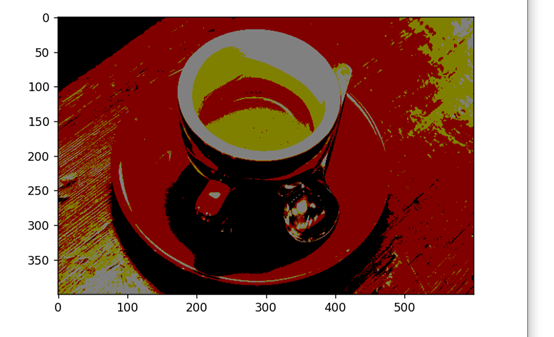5.my thinking
量化和采样是类似的，都是对连续量的离散化处理，都是为了让图像能够被计算机处理。
参考：《数字图像处理与python实现》
展开全文计算机视觉 python
• 本篇文章主要总结了图像的采样以及量化的原理以及操作，在此基础上添加了图像的马赛克处理以及图像金子塔，其中参考的一些文章的链接也添加了进去，如果需要更深入的了解和学习可以点进去学习
对于信号的采样可以参考我之前的文章：数字信号处理 2.1 — 采样
在本篇文章中绘图使用到了 matplotlib 库，需要了解学习可以参考我之前写的用来总结这个绘图库的文章：Python 绘图库 Matplotlib
目录
1. 图像量化与采样处理
（1）基本概念
（2）量化操作
（2）采样操作
2. 图像金字塔
（1）基本概念
（2）操作实现
高斯金子塔下采样
拉普拉斯金子塔恢复原图像
3. 局部马赛克处理
（1）实现原理
（2）实战操作
结束语

1. 图像量化与采样处理
经典开头读取图像信息：
"""
Author:XiaoMa
date:2021/10/22
"""
#调用所需的包
import matplotlib.pyplot as plt
import cv2
import numpy as np

#读取原始图像的信息
img0 = cv2.imread('E:\From Zhihu\For the desk\ZHM.jpeg')   #读取图像
img1 = cv2.resize(img0, fx = 0.5, fy = 0.5, dsize = None)  #调整图像大小
img2 = cv2.cvtColor(img1, cv2.COLOR_BGR2GRAY)              #将图像转化为灰度图像
height = img1.shape                                     #shape 图像第一维度，高度
width = img1.shape                                      #shape 图像第二维度，宽度
print(img1.shape)
print(width, height)
cv2.namedWindow("W0")
cv2.imshow("W0", img1)
cv2.waitKey(delay = 0)（1）基本概念
此处参考：知乎文章
把一幅图像表示为二元函数形式：f(x, y)，那么该函数在不同的坐标点有不同的幅度值，而这些幅度值是连续的，无法使用计算机进行处理，所以我们需要对幅度值进行离散化（数字化），这个过程即为图像量化处理，一般常见的量化方式是将图像用黑白两种颜色表示，称为二值图像
在之前的文章中提到一幅图像可以表示为在 x 轴和 y 轴上连续的信号，但计算机无法处理连续信号，所以我们需要将图像在这两个维度进行离散化处理，该过程就称为图像的采样处理，即对图像的坐标轴进行数字化
（2）量化操作
具体代码如下，都已经添加了注释，理解起来应该没问题，如果有我注释的不清楚的地方可以在评论区提出来，我们一起探讨学习：
"""
Author:XiaoMa
date:2021/10/22
"""
#调用所需的包
import matplotlib.pyplot as plt
import cv2
import numpy as np

#读取原始图像的信息
img0 = cv2.imread('E:\From Zhihu\For the desk\ZHM.jpeg')   #读取图像
img1 = cv2.resize(img0, fx = 0.5, fy = 0.5, dsize = None)  #调整图像大小
img2 = cv2.cvtColor(img1, cv2.COLOR_BGR2GRAY)              #将图像转化为灰度图像
height = img1.shape                                     #shape 图像第一维度，高度
width = img1.shape                                      #shape 图像第二维度，宽度
print(img1.shape)
print(width, height)
cv2.namedWindow("W0")
cv2.imshow("W0", img2)
cv2.waitKey(delay = 0)

#创建和原始图像同等大小的矩阵
img3 = np.zeros((width, height, 3), np.uint(8))
img4 = np.zeros((width, height, 3), np.uint(8))
img5 = np.zeros((width, height, 3), np.uint(8))
img6 = np.zeros((width, height, 3), np.uint(8))
img7 = np.zeros((width, height, 3), np.uint(8))

#对原始图像矩阵的值进行操作
img3 = np.uint8(img2/4) * 4
img4 = np.uint8(img2/16) * 16
img5 = np.uint8(img2/32) * 32
img6 = np.uint8(img2/64) * 64
img7 = np.uint8(img2 >= 128) * 128

plt.rcParams['font.family'] = 'SimHei'       #将全局中文字体改为黑体
plt.rcParams['axes.unicode_minus'] = False   #正常表示负号

#显示得到的图像
title = ['原始图像', '量化为64份', '量化为16份', '量化为8份', '量化为4份', '量化为2份']    #子图标题
img = [img2, img3, img4, img5, img6, img7]
for i in range(6):
plt.subplot(2, 3, i + 1)   #python 列表从0开始计数，所以此处 i+1
plt.imshow(img[i], 'gray')
plt.title(title[i])
plt.xticks([]),plt.yticks([])
plt.savefig('E:\From Zhihu\For the desk\ZHM1.jpeg')
plt.show()

然后得到的量化后的图像如下：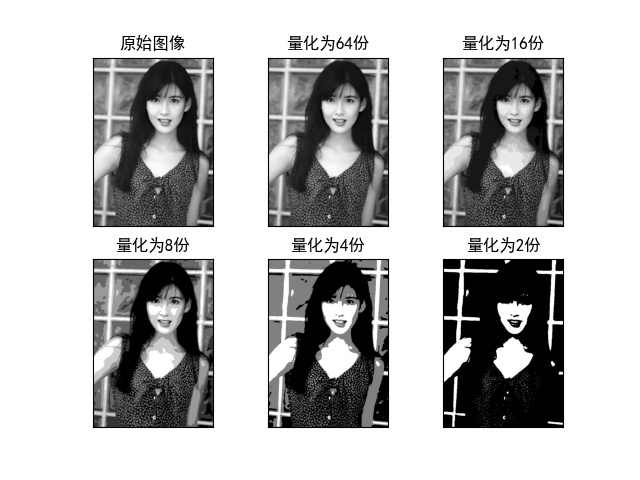（2）采样操作
具体代码如下：
"""
Author:XiaoMa
date:2021/10/22
"""
#调用所需的包
import matplotlib.pyplot as plt
import cv2
import numpy as np

#读取原始图像的信息
img0 = cv2.imread('E:\From Zhihu\For the desk\ZHM.jpeg')   #读取图像
img1 = cv2.resize(img0, fx = 0.5, fy = 0.5, dsize = None)  #调整图像大小
img2 = cv2.cvtColor(img1, cv2.COLOR_BGR2GRAY)              #将图像转化为灰度图像

height = img1.shape                                     #shape 图像第一维度，高度
width = img1.shape                                      #shape 图像第二维度，宽度
print(img1.shape)
print(width, height)
cv2.namedWindow("W0")
cv2.imshow("W0", img1)
cv2.waitKey(delay = 0)

plt.rcParams['font.family'] = 'SimHei'       #将全局中文字体改为黑体

img8 = img2[0:-1:2, 0:-1:2]
#img8_1 = cv2.resize(img8, dsize = None, fx = 2, fy = 2)   #这个注释先不管，后面解释
img9 = img2[0:-1:4, 0:-1:4]
#img9_1 = cv2.resize(img9, dsize = None, fx = 4, fy = 4)
img10 = img2[0:-1:8, 0:-1:8]
#img10_1 = cv2.resize(img10, dsize = None, fx = 8, fy = 8)
img11 = img2[0:-1:16, 0:-1:16]
#img11_1 = cv2.resize(img11, dsize = None, fx = 16, fy = 16)
titles = ['原始图像', '256*256', '128*128', '64*64', '32*32', '16*16']
image = [img1, img2, img8, img9, img10, img11]      #此处一定要注意不加引号，别问我为什么知道，心累

for j in range(6):
plt.subplot(2, 3, j + 1)
if j == 0:
plt.imshow(image[j])
else:
plt.imshow(image[j], 'gray')
plt.title(titles[j])
plt.xticks([]), plt.yticks([])
plt.savefig('E:\From Zhihu\For the desk\ZHM2.jpeg')
plt.show()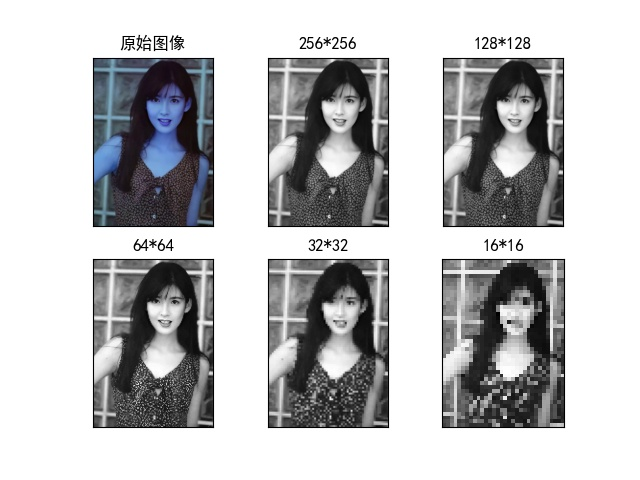得到的图像如上，但是第一幅图是不是很怪？其实原因在前面讲过的：

OpenCV 对于图像的处理方式是 BGR 的，而对于 matplotlib 来说是 RGB 的，所以要将通道顺序进行修改：

只要加上这一句代码就可以了，添加的位置是在组成列表之前就行
img_rgb = cv2.cvtColor(img1, cv2.COLOR_BGR2RGB)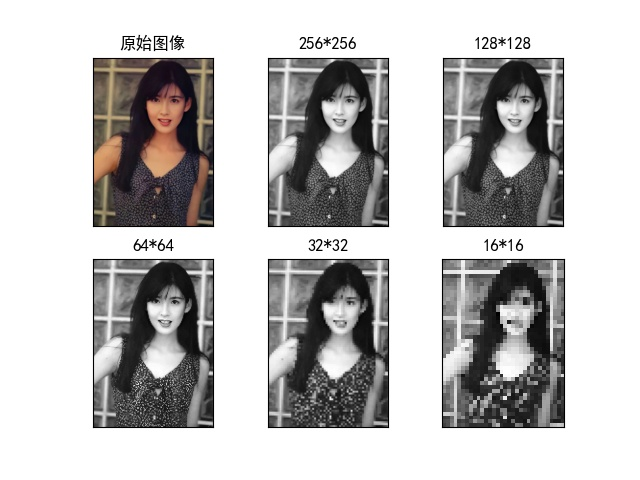关于前面的代码中的注释部分：图像经过采样之后尺寸大小发生变化，对其尺寸进行调整使其能够正常显示（当然这只会在单独显示图像时用到）
如：未改变尺寸前的 16*16 图像如下（在原图左上角）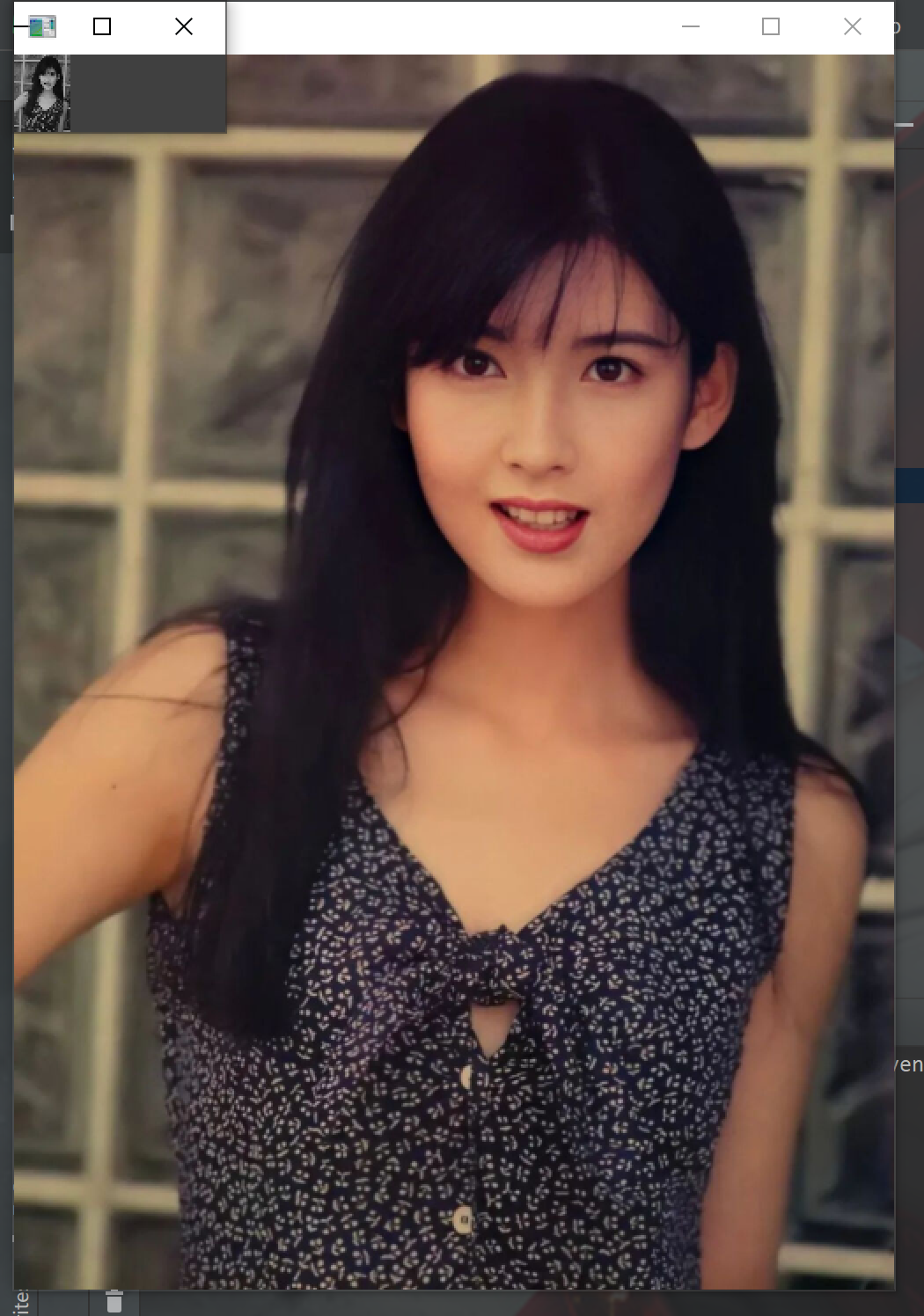经过改变后的图像如下：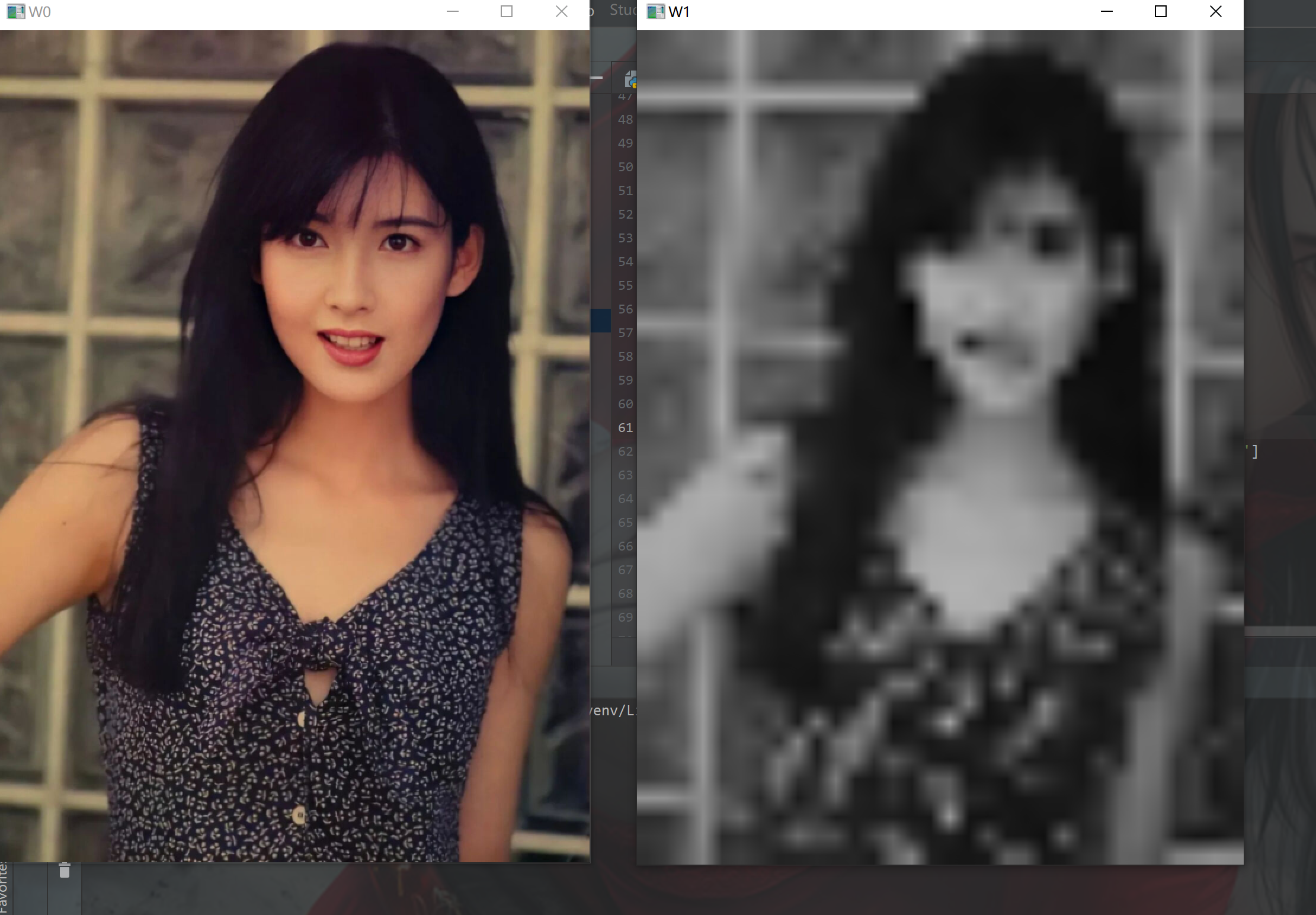2. 图像金字塔
（1）基本概念
概念这方面参考的文章：OpenCV（23）---图像金字塔
图像金字塔是由一副图像的多个不同分辨率的子图所构成的图像集合。该组图像是由单个图像通过不断地降低采样所产生的，最小的图像可能仅仅只有一个像素点。如下图所示，分辨率从低到高，逐渐降低的图像集合。（该段全部摘自上面的文章）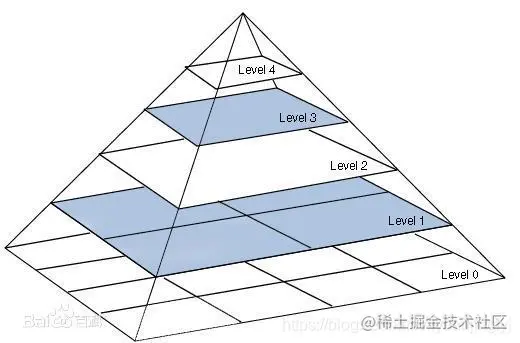下面说说我的理解：在图像的采样的介绍中我们得到了五张经过采样后的图像，而它们的分辨率都是不同的， 因为在采样的过程中总会失去一些信息，图像中失去的是像素点（这方面不理解可以参考文章头粘贴的关于采样的文章），然后将不同分辨率的图像按照上图中的金子塔从大到小排列上去，就是图像金子塔了就拿上图举例，所谓的图像金子塔就是将第二幅图放在 Level0 层，将第三幅放在 Level1 层，以此类推，得到最终的图像
（2）操作实现
高斯金子塔下采样
#调用所需的包
import matplotlib.pyplot as plt
import cv2

#读取原始图像的信息
img0 = cv2.imread('E:\From Zhihu\For the desk\ZHM.jpeg')   #读取图像
img1 = cv2.resize(img0, fx = 0.5, fy = 0.5, dsize = None)  #调整图像大小
img2 = cv2.cvtColor(img1, cv2.COLOR_BGR2GRAY)              #将图像转化为灰度图像

img12 = cv2.pyrDown(img1)                                  #高斯滤波下采样得到高斯金字塔
cv2.namedWindow("W1")
cv2.imshow("W1", img12)
cv2.waitKey(delay = 0)将其与原图像对比：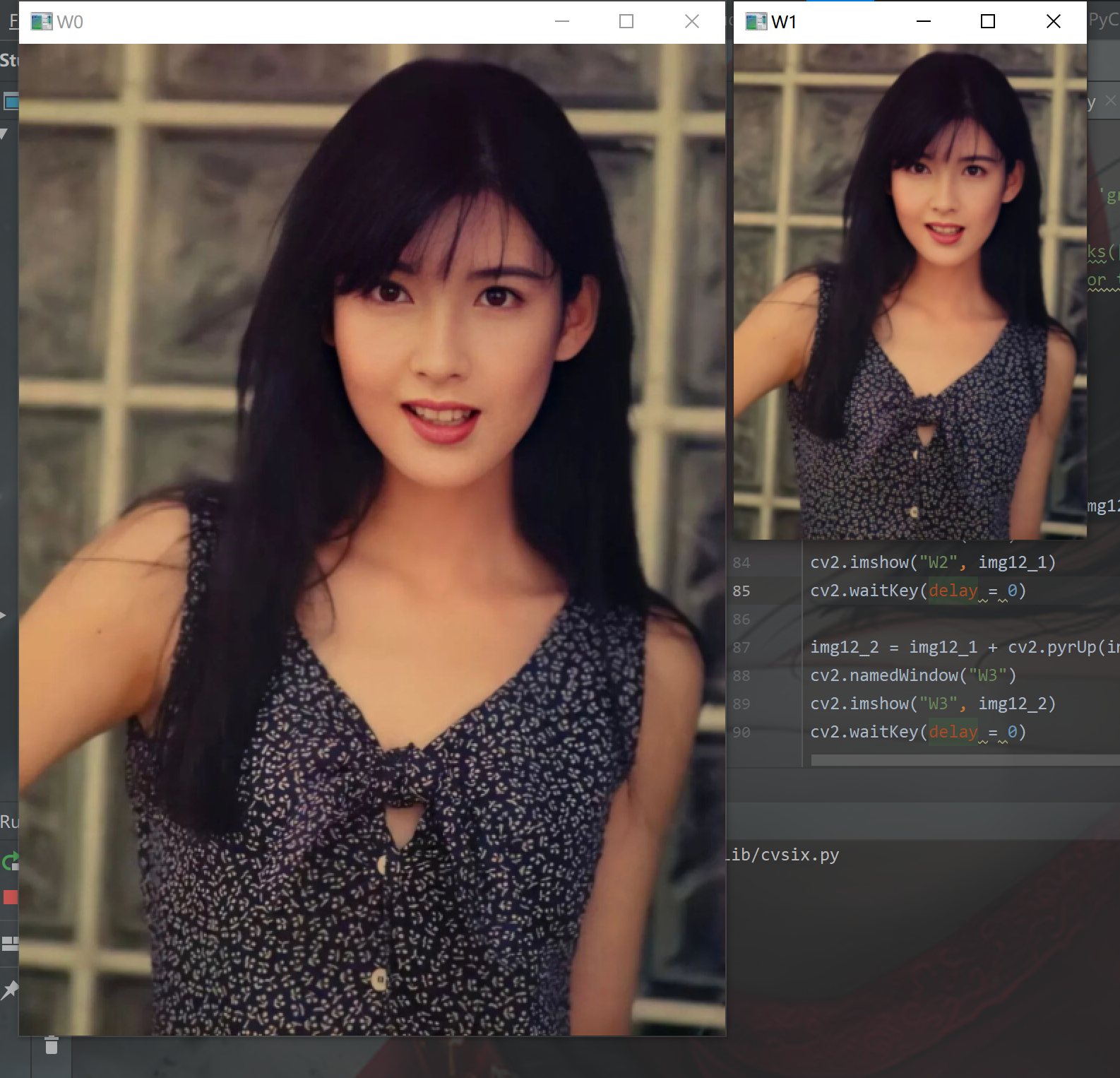可以看出，经过降采样得到的图像与原图像对比最主观的变化是尺寸大小的变化，当然尺寸大小也可以通过参数进行设置，其实就是对采样率的设置了
拉普拉斯金子塔恢复原图像
通过高斯金子塔下采样得到的图像没办法通过高斯上采样恢复出来，因为按照采样的意义来说已经丢失了那部分的像素点，没办法凭空出现，但丢失的那些信息，刚好就构成了拉普拉斯金字塔，将高斯金字塔加进去就能复原出原图像了
#调用所需的包
import matplotlib.pyplot as plt
import cv2

#读取原始图像的信息
img0 = cv2.imread('E:\From Zhihu\For the desk\ZHM.jpeg')   #读取图像
img1 = cv2.resize(img0, fx = 0.5, fy = 0.5, dsize = None)  #调整图像大小
img2 = cv2.cvtColor(img1, cv2.COLOR_BGR2GRAY)              #将图像转化为灰度图像

img12 = cv2.pyrDown(img2)                                  #高斯滤波下采样得到高斯金字塔
cv2.namedWindow("W1")
cv2.imshow("W1", img12)
cv2.waitKey(delay = 0)

img12_1 = img1 - cv2.pyrUp(img12)                           #得到拉普拉斯金字塔
cv2.namedWindow("W2")
cv2.imshow("W2", img12_1)
cv2.waitKey(delay = 0)

img12_2 = img12_1 + cv2.pyrUp(img12)                        #恢复原图像
cv2.namedWindow("W3")
cv2.imshow("W3", img12_2)
cv2.waitKey(delay = 0)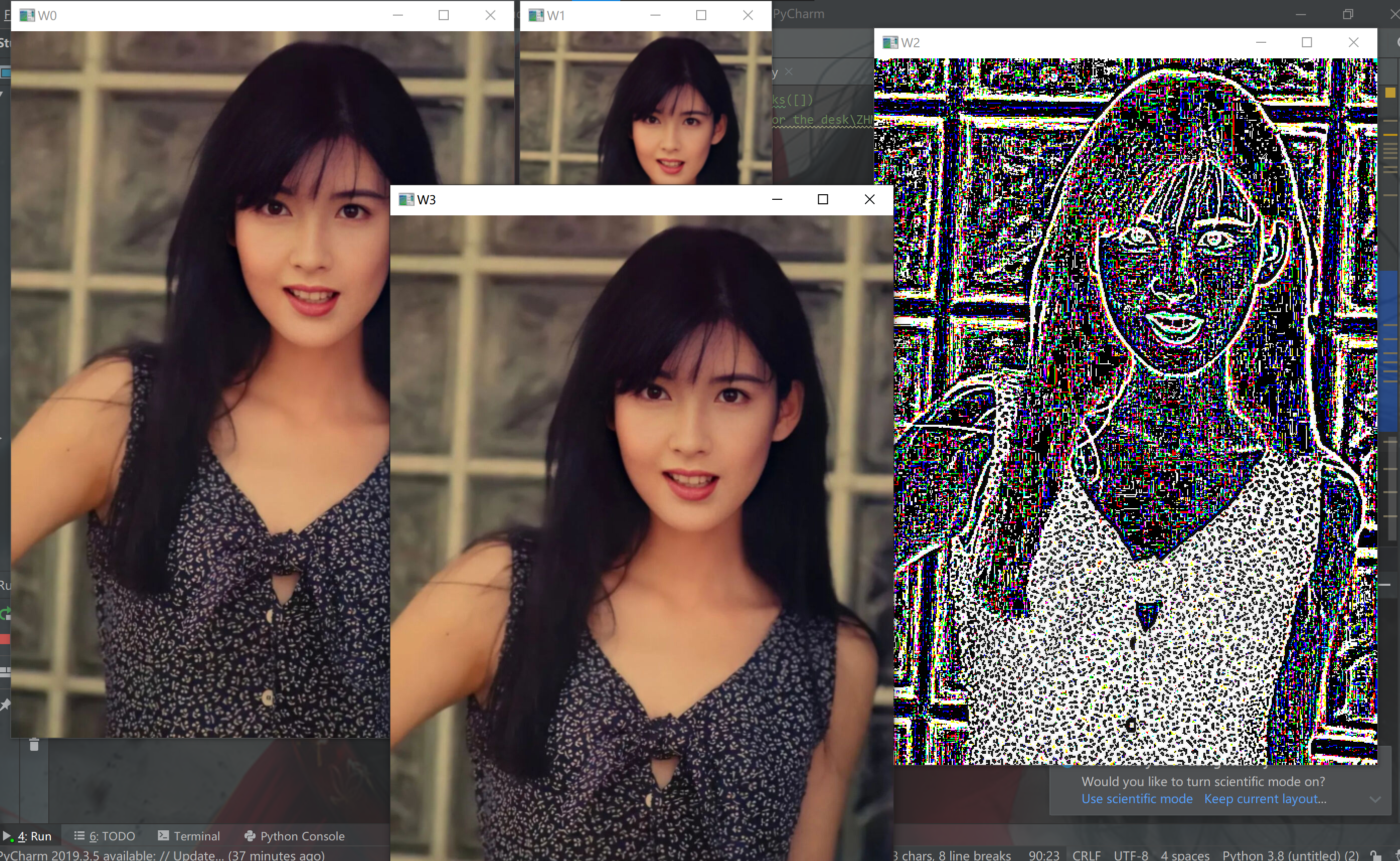W0 中的是原图，W1 是经过下采样得到的高斯金字塔，W2 是拉普拉斯金字塔，W3 是恢复出来的原图像
3. 局部马赛克处理
该段参考文章：图片处理之马赛克
（1）实现原理
a:确定需要生成马赛克的目标区域
b:将目标区域分为许多同等大小的，为了打码效果尽可能的好，划分的数目一般不能过多或过少
c:在划分的区域中随机选择一个像素点，用这个像素点代替所有该区域中的像素点
（2）实战操作
import cv2

#读取原始图像的信息
img0 = cv2.imread('E:\From Zhihu\For the desk\ZHM.jpeg')   #读取图像
img1 = cv2.resize(img0, fx = 0.5, fy = 0.5, dsize = None)  #调整图像大小
img2 = cv2.cvtColor(img1, cv2.COLOR_BGR2GRAY)              #将图像转化为灰度图像

height = img1.shape                                     #shape 图像第一维度，高度
width = img1.shape                                      #shape 图像第二维度，宽度
print(img1.shape)
print(width, height)
cv2.namedWindow("W0")
cv2.imshow("W0", img1)
cv2.waitKey(delay = 0)

for i in range(200, 400, 10):           #生产马赛克的目标区域(对图像高度)
for j in range(200, 400, 10):       #生成马赛克的目标区域(对图像宽度)
for m in range(0, 10):      #区域大小为 10 * 10
for n in range(0, 10):
img1[i+m, j+n] = img1[i, j]   #用该区域的第一个像素点替换掉其他的像素点
cv2.namedWindow("W4")
cv2.imshow("W4", img1)
cv2.waitKey(delay = 0)
得到图像如下：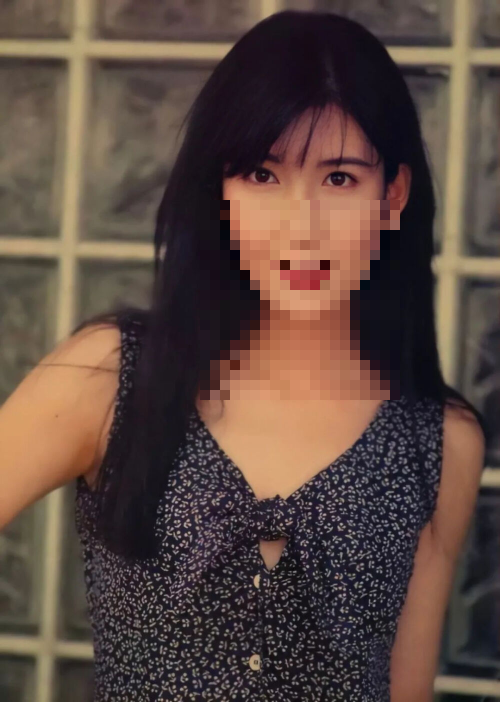结束语
本篇文章主要总结了图像的采样以及量化的原理以及操作，在此基础上添加了图像的马赛克处理以及图像金子塔，其中参考的一些文章的链接也添加了进去，如果需要更深入的了解和学习可以点进去学习
展开全文计算机视觉 python opencv 1024程序员节
• Exoquant 0.1.0 Exoquant是用Rust编写的高质量图像量化库，具有用于基本颜色量化的代码，K-Means调色板Exoquant 0.1.0 Exoquant是用Rust编写的高质量图像量化库，具有用于基本颜色量化的代码， K-Means调色板优化...
• 使用matlab语言，对图像进行处理，实现图像灰度量化操作，改变图像灰度级。
• 图像量化器 脚本可将图像量化为调色板。 受启发。 默认情况下，它将对图像应用（线性）预模糊以减少噪声，然后将每个像素量化为Monokai配色方案中的一种颜色。 跑步 需要才能运行 jbang ImageQuantizer.java input...Java
• 对于lena图像量化，以及利用Lloyd Max函数量化，有文档，有代码，运行通过的，平台式matlab！
• 图像量化函数——matlab版 代码片. // An highlighted block function ouput_img = quantize(input_img,level) % quantize 图像量化函数 % input_img：输入图像 % level: 给定的图像灰度级数 % ouput_img：输出图像 ...


图像量化函数——matlab版

代码片.
// An highlighted block
function ouput_img = quantize(input_img,level)
%	quantize 图像量化函数
%   input_img：输入图像
%   level: 给定的图像灰度级数
%   ouput_img：输出图像
src = double(input_img);% 将输入图像转为double型，便于后续取整操作
[m, n] = size(src);
k = level/256;  %计算量化前后的比值
dst = zeros(m,n,'uint8'); % 建立8为无符号的图像
res = zeros(m,n,'uint8');

for i = 1 : n
for j = 1 : m
dst(j,i) = fix(k*src(j,i)); %向零取整
end
end
% 寻找图像中的最大、最小灰度值
max_dst = 0;
min_dst = 255;
for i = 1 : n
for j = 1 : m
if dst(j,i)> max_dst
max_dst = dst(j,i);
end
if dst(j,i)< min_dst
min_dst = dst(j,i);
end
end
end

%将图像灰度值映射到0~255之间
for i = 1 : n
for j = 1 : m
res(j,i) = round(dst(j,i)*(255/(max_dst - min_dst)));
end

end
ouput_img = res;
end


展开全文matlab 计算机视觉
• import numpy as np import scipy.misc as sm import scipy.ndimage as sn import sklearn.cluster as sc import matplotlib.pyplot as...# 通过K均值聚类量化图像中的颜色 def quant(image, n_clusters): x = imag...
import numpy as np
import scipy.misc as sm
import scipy.ndimage as sn
import sklearn.cluster as sc
import matplotlib.pyplot as mp

# 通过K均值聚类量化图像中的颜色
def quant(image, n_clusters):
x = image.reshape(-1, 1)
model = sc.KMeans(n_clusters=n_clusters)
model.fit(x)
y = model.labels_
centers = model.cluster_centers_.ravel()
return centers[y].reshape(image.shape)

quant4 = quant(original, 4)
quant3 = quant(original, 3)
quant2 = quant(original, 2)
mp.figure('Image Quant', facecolor='lightgray')
mp.subplot(221)
mp.title('Original', fontsize=16)
mp.axis('off')
mp.imshow(original, cmap='gray')
mp.subplot(222)
mp.title('Quant-4', fontsize=16)
mp.axis('off')
mp.imshow(quant4, cmap='gray')
mp.subplot(223)
mp.title('Quant-3', fontsize=16)
mp.axis('off')
mp.imshow(quant3, cmap='gray')
mp.subplot(224)
mp.title('Quant-2', fontsize=16)
mp.axis('off')
mp.imshow(quant2, cmap='gray')
mp.tight_layout()
mp.show()

展开全文• 该算法将 32 位 RGBA 图像量化为 8 位颜色。 通过调整采样因子，该算法可以缓慢地产生极高质量的图像，或者在合理的时间内产生良好的图像。 采样因子为 10 可显着提高速度，但质量损失很小。 import "dart:html"; ...Dart
• 前面一篇文章我讲解了基于K-Means聚类的图像分割或量化处理，但突然发现世面上的文章讲解图像量化和采样代码的很缺乏，因此结合2015年自己的一篇文章，补充相关知识供同学们学习。基础性文章，希望对你有所帮助。...K-Means聚类
• 使用近红外图像量化植物健康和光合活动 语言：C++ 日期：2014 年 12 月 17 日 添加一名作者 参考： 用法： 该程序将拍摄 PNG 格式的红外线图像（来自带有蓝色滤镜的 NoIR 相机）。 它将可选地输出标准化差异...C++
• 使用聚类算法 对图像量化 图像量化 将图像 0-255 变成设定的值类型种类 """ import numpy as np import cv2 import sklearn.cluster as sc import matplotlib.pyplot as mp # 加载图片 original = cv2.imread("./...
• 行业分类-物理装置-图像量化方法、计算机设备和存储介质.zip
• 以JPEG图像为载体，探讨了一种修改JPEG图像量化表的信息隐藏方法。由于JPEG图像编码信息损失主要发生在量化过程，因此，首先通过计算JPEG图像量化后的DCT系数和反量化后的DCT系数之间的差异寻找信息隐藏的最佳位置；...
• 最近在给搞图像去雾的师兄打杂，整理几个评价图像的指标：PSNR,MSE,SSIM,WSNR PSNR,MSE,SSIM:　http://www.voidcn.com/blog/xiaohaijiejie/article/p-3086738.html PSNR,MSE： ...
• 提出了一种基于Fisher判据的自适应彩色图像量化算法。首先用八叉树算法把原始图像量化为256种颜色，然后根据人类的视觉特性，参照NBS距离与人类视觉对颜色差别的定量关系，自动确定初始聚类中心及聚类数目，在此基础...
• 高分辨率透射电镜碳烟图像量化分析方法研究，石晓龙，沈涛，为了减少柴油颗粒物污染，则必须研究柴油机中排气颗粒物即碳烟的生成机理。高分辨率透射电镜拍摄的碳烟图像具有所见即所得的直观
• 它还对某些图像类型使用高性能的直接像素访问，从而减少了内存占用并提高了吞吐量。 基准测试 与现有的量化库相比，go-quantize的执行速度明显更快： # bench/bench_test.go BenchmarkQuantize-8 50 20070550 ...Go
• matlab开发-flxtblwriter图像量化器。与“write_flex_material_table.m”一起使用
•研究论文
• p5.j​​s量化图像算法 Conheçao P5.js p5.j​​s和uma biblioteca JavaScript的标准注释，包括注释，包括艺术家，设计师，教育工作者，合格人士和比索！ 安装项目 git clone ...JavaScript
• 目的 利用粒子群优化算法和K-均值方法研究彩色图像量化问题。方法 针对K-均值聚类量化算法对初始值比较敏感，易陷入局部极小值从而使得算法得不到全局最优解，为局部搜索算法，以及粒子群优化算法是一种全局寻优...
• 能够检测出JPEG图像量化表，和质量压缩因数等各方面信息....